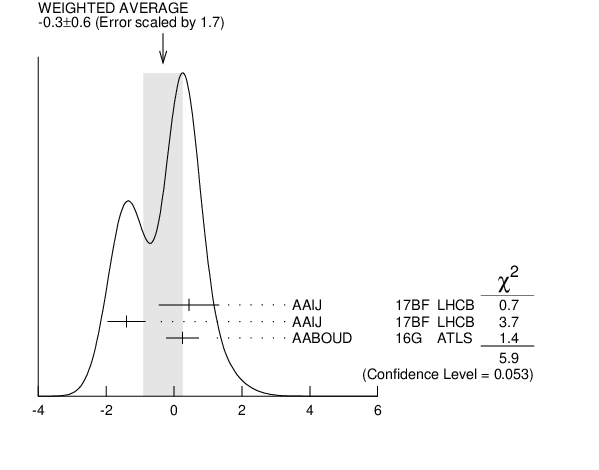#### A$_{P}({{\boldsymbol B}^{0}}$)

A$_{P}({{\mathit B}^{0}}$) = [${\mathit \sigma (}{{\overline{\mathit B}}^{0}}{)}$ $−$ ${\mathit \sigma (}{{\mathit B}^{0}}{)}$] $/$ [${\mathit \sigma (}{{\overline{\mathit B}}^{0}}{)}$ $+$ ${\mathit \sigma (}{{\mathit B}^{0}}{)}$]
VALUE ($10^{-2}$) DOCUMENT ID TECN  COMMENT
 $\bf{ -0.3 \pm0.6}$ OUR AVERAGE  Error includes scale factor of 1.7. See the ideogram below.
$0.44$ $\pm0.88$ $\pm0.11$ 1
 2017 BF
LHCB ${{\mathit p}}{{\mathit p}}$ at 7 TeV
$-1.40$ $\pm0.55$ $\pm0.10$ 1
 2017 BF
LHCB ${{\mathit p}}{{\mathit p}}$ at 8 TeV
$0.25$ $\pm0.48$ $\pm0.05$ 2
 2016 G
ATLS ${{\mathit p}}{{\mathit p}}$ at 7, 8 TeV
• • We do not use the following data for averages, fits, limits, etc. • •
$-0.35$ $\pm0.76$ $\pm0.28$ 3
 2014 BP
LHCB Repl. by AAIJ 2017BF, ${{\mathit p}}{{\mathit p}}$ at 7 TeV
 1 AAIJ 2017BF uses ${{\mathit B}^{0}}$ $\rightarrow$ ${{\mathit J / \psi}}{{\mathit K}^{*0}}$ decays with ${{\mathit B}^{0}}$ transverse momenta $p_T$ and rapidities y in the region of 0 $<$ $p_T$ $<$ 30 GeV/c and 2.1 $<$ y $<$ 4.5.
 2 Based on time-dependent analysis of ${{\mathit B}^{0}}$ $\rightarrow$ ${{\mathit J / \psi}}{{\mathit K}^{*0}}$ decay in kinematic range $p_T$ $>$ 10 GeV/c and $\vert \eta \vert$ $<$ 2.5.
 3 Based on time-dependent analysis of ${{\mathit B}^{0}}$ $\rightarrow$ ${{\mathit J / \psi}}{{\mathit K}^{*0}}$ and ${{\mathit B}^{0}}$ $\rightarrow$ ${{\mathit D}^{-}}{{\mathit \pi}^{+}}$ in kinematic range 4 $<$ $p_T$ $<$ 30 GeV/c and 2.5 $<\eta$ $<$ 4.5.A$_{P}({{\mathit B}^{0}}$) ($10^{-2}$)
References:
 AAIJ 2017BF
PL B774 139 Measurement of ${{\mathit B}^{0}}$, ${{\mathit B}_{{s}}^{0}}$, ${{\mathit B}^{+}}$ and ${{\mathit \Lambda}_{{b}}^{0}}$ Production Asymmetries in 7 and 8 TeV Proton-Proton Collisions
 AABOUD 2016G
JHEP 1606 081 Measurement of the Relative width Difference of the ${{\mathit B}^{0}}−{{\overline{\mathit B}}^{0}}$ System with the ATLAS Detector
 AAIJ 2014BP
PL B739 218 Measurement of the ${{\overline{\mathit B}}^{0}}−{{\mathit B}^{0}}$ and ${{\overline{\mathit B}}_{{s}}^{0}}−{{\mathit B}_{{s}}^{0}}$ Production Asymmetries in ${{\mathit p}}{{\mathit p}}$ Collisions at $\sqrt {s }$ = 7 TeV